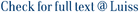We tackle the problem of counting the number qk of k-cliques in large-scale graphs, for any constant k ≥ 3. Clique counting is essential in a variety of applications, including social network analysis. Our algorithms make it possible to compute qk for several real-world graphs and shed light on its growth rate as a function of k. Even for small values of k, the number qk of k-cliques can be in the order of tens or hundreds of trillions. As k increases, different graph instances show different behaviors: while on some graphs qk+1 &lt; qk, on other benchmarks qk+1 qk, up to two orders of magnitude in our observations. Graphs with steep clique growth rates represent particularly tough instances in practice. Due to the computationally intensive nature of the clique counting problem, we settle for parallel solutions in the MapReduce framework, which has become in the last few years a de facto standard for batch processing of massive datasets. We give both theoretical and experimental contributions. On the theory side, we design the first exact scalable algorithm for counting (and listing) k-cliques in MapReduce. Our algorithm uses O(m3/2) total space and O(mk/2) work, where m is the number of graph edges. This matches the best-known bounds for triangle listing when k = 3 and is work optimal in the worst case for any k, while keeping the communication cost independent of k. We also design sampling-based estimators that can dramatically reduce the running time and space requirements of the exact approach, while providing very accurate solutions with high probability. We then assess the effectiveness of different clique counting approaches through an extensive experimental analysis over the Amazon EC2 platform, considering both our algorithms and their state-of-the-art competitors. The experimental results clearly highlight the algorithm of choice in different scenarios and prove our exact approach to be the most effective when the number of k-cliques is large, gracefully scaling to nontrivial values of k even on clusters of small/medium size. Our approximation algorithms achieve extremely accurate estimates and large speedups, especially on the toughest instances for the exact algorithms

Clique counting in MapReduce: Algorithms and experiments / Finocchi, Irene; Finocchi, Marco; Fusco, EMANUELE GUIDO. - In: ACM JOURNAL OF EXPERIMENTAL ALGORITHMICS. - ISSN 1084-6654. - 20:1(2015), pp. 1-20. [10.1145/2794080]

### Clique counting in MapReduce: Algorithms and experiments

#### Abstract

We tackle the problem of counting the number qk of k-cliques in large-scale graphs, for any constant k ≥ 3. Clique counting is essential in a variety of applications, including social network analysis. Our algorithms make it possible to compute qk for several real-world graphs and shed light on its growth rate as a function of k. Even for small values of k, the number qk of k-cliques can be in the order of tens or hundreds of trillions. As k increases, different graph instances show different behaviors: while on some graphs qk+1 < qk, on other benchmarks qk+1 qk, up to two orders of magnitude in our observations. Graphs with steep clique growth rates represent particularly tough instances in practice. Due to the computationally intensive nature of the clique counting problem, we settle for parallel solutions in the MapReduce framework, which has become in the last few years a de facto standard for batch processing of massive datasets. We give both theoretical and experimental contributions. On the theory side, we design the first exact scalable algorithm for counting (and listing) k-cliques in MapReduce. Our algorithm uses O(m3/2) total space and O(mk/2) work, where m is the number of graph edges. This matches the best-known bounds for triangle listing when k = 3 and is work optimal in the worst case for any k, while keeping the communication cost independent of k. We also design sampling-based estimators that can dramatically reduce the running time and space requirements of the exact approach, while providing very accurate solutions with high probability. We then assess the effectiveness of different clique counting approaches through an extensive experimental analysis over the Amazon EC2 platform, considering both our algorithms and their state-of-the-art competitors. The experimental results clearly highlight the algorithm of choice in different scenarios and prove our exact approach to be the most effective when the number of k-cliques is large, gracefully scaling to nontrivial values of k even on clusters of small/medium size. Our approximation algorithms achieve extremely accurate estimates and large speedups, especially on the toughest instances for the exact algorithms
##### Scheda breve Scheda completa Scheda completa (DC)Clique listing; graph algorithms; MapReduce; parallel algorithms; ex- perimental algorithmics
Clique counting in MapReduce: Algorithms and experiments / Finocchi, Irene; Finocchi, Marco; Fusco, EMANUELE GUIDO. - In: ACM JOURNAL OF EXPERIMENTAL ALGORITHMICS. - ISSN 1084-6654. - 20:1(2015), pp. 1-20. [10.1145/2794080]
File in questo prodotto:
File
Finocchi_Clique-Counting_2015.pdf

Solo gestori archivio

Tipologia: Versione dell'editore
Licenza: DRM non definito
Dimensione 467.32 kB
Utilizza questo identificativo per citare o creare un link a questo documento: `http://hdl.handle.net/11385/192593`
•29
•ND